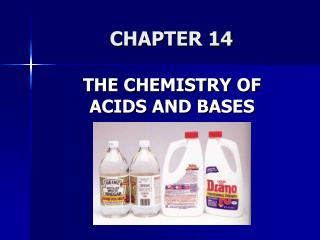DownloadDownload PresentationCHAPTER 14

# CHAPTER 14

Download Presentation## CHAPTER 14

- - - - - - - - - - - - - - - - - - - - - - - - - - - E N D - - - - - - - - - - - - - - - - - - - - - - - - - - -
##### Presentation Transcript

1. CHAPTER 14 THE CHEMISTRY OF ACIDS AND BASES

2. "ACID" Latin word acidus, meaning sour. (lemon)

3. "ALKALI" Water solutions feel slippery and taste bitter (soap).

4. ACID-BASETHEORIES

5. Arrhenius Definition acid--donates a hydrogen ion (H+) in water base--donates a hydroxide ion in water (OH-) This theory was limited to substances with those "parts"; ammonia is a MAJOR exception!

6. Bronsted-Lowry Definition acid--donates a proton in water base--accepts a proton in water This theory is better; it explains ammonia as a base! This is the main theory that we will use for our acid/base discussion.

7. Lewis Definition acid--accepts an electron pair base--donates an electron pair This theory explains alltraditional acids and bases + a host of coordination compounds and is used widely in organic chemistry. Uses coordinate covalent bonds

8. The Bronsted-Lowry Concept of Acids and Bases Using this theory, you should be able to write weak acid/base dissociation equations and identify acid, base, conjugate acid and conjugate base.

9. Conjugate Acid-Base Pair A pair of compounds that differ by the presence of one H+ unit. This idea is critical when it comes to understanding buffer systems.

10. Acids donate a proton (H+)

11. Neutral Compound HNO3 + H2O  H3O+ + NO3- acid base CA CB

12. Cation NH4+ + H2O  H3O+ + NH3 acid base CA CB

13. Anion H2PO4- + H20  H3O+ + HPO42- acid base CA CB

14. In each of the acid examples---notice the formation of H3O+ This species is named the hydronium ion. It lets you know that the solution is acidic!

15. Hydronium, H3O+ --H+ riding piggy-back on a water molecule. Water is polar and the + charge of the naked proton is greatly attracted to Mickey's chin!)

16. Bases accept a proton (H+)

17. Neutral Compound NH3 + H2O  NH4+ + OH- base acid CA CB

18. Anion CO32- + H2O  HCO3- + OH- base acid CA CB

19. Anion PO43- + H2O  HPO42- + OH- base acid CA CB

20. In each of the basic examples-- notice the formation of OH- -- this species is named the hydroxide ion. It lets you know that the solution is basic!

21. Exercise 1 In the following reaction, identify the acid on the left and its CB on the right. Similarly identify the base on the left and its CA on the right. HBr + NH3 NH4+ + Br-

22. What is the conjugate base of H2S? What is the conjugate acid of NO3-?

23. ACIDS ONLY DONATE ONE PROTON AT A TIME!!!

24. monoprotic--acids donating one H+ (ex. HC2H3O2) diprotic--acids donating two H+'s (ex. H2C2O4) polyprotic--acids donating many H+'s (ex. H3PO4)

25. Polyprotic Bases accept more than one H+ anions with -2 and -3 charges (example: PO43- ; HPO42-)

26. Amphiprotic or Amphoteric molecules or ions that can behave as EITHER acids or bases: water, anions of weak acids (look at the examples above—sometimes water was an acid, sometimes it acted as a base)

27. Exercise 2 Acid Dissociation (Ionization) Reactions Write the simple dissociation (ionization) reaction (omitting water) for each of the following acids.

28. a. Hydrochloric acid (HCl) b. Acetic acid (HC2H3O2) c. The ammonium ion (NH4+) d. The anilinium ion (C6H5NH3+) e. The hydrated aluminum(III) ion [Al(H2O)6]3+

29. Solution A: HCl(aq) -> H+(aq) + Cl-(aq) B: HC2H3O2(aq)  H+(aq) + C2H3O2-(aq) C: NH4+(aq)  H+(aq) + NH3(aq)

30. Solution, cont. D: C6H5NH3+(aq)  H+(aq) + C6H5NH2(aq) E: Al(H2O)63+(aq)  H+(aq) + Al(H2O)5OH2+(aq)

31. Relative Strengths of Acids and Bases Strength is determined by the position of the "dissociation" equilibrium.

32. Strong acids/Strong bases dissociate completely in water have very large K values

33. Weak acids/Weak bases dissociate only to a slight extent in water dissociation constant is very small

34. Strong Weak

35. Do Not confuse concentration with strength!

36. Strong Acids Hydrohalic acids: HCl, HBr, HI Nitric: HNO3 Sulfuric: H2SO4 Perchloric: HClO4

37. The more oxygen present in the polyatomic ion, the stronger its acid WITHIN that group.

38. Strong Bases Hydroxides OR oxides of IA and IIA metals Solubility plays a role (those that are very soluble are strong!)

39. Weak Acids and Bases - Equilibrium expressions The vast majority of acid/bases are weak. Remember, this means they do not ionize much.

40. The equilibrium expression for acids is known as the Ka (the acid dissociation constant). It is set up the same way as in general equilibrium.

41. Many common weak acids are oxyacids, like phosphoric acid and nitrous acid.

42. Other common weak acids are organic acids,those that contain a carboxyl group COOH group like acetic acid and benzoic acid.

43. For Weak Acid Reactions: HA + H2O  H3O+ + A- Ka = [H3O+][A-] < 1 [HA]

44. Write the Ka expression for acetic acid using Bronsted-Lowry. (Note: Water is a pure liquid and is thus, left out of the equilibrium expression.)

45. Weak bases (bases without OH-) react with water to produce a hydroxide ion.

46. Common examples of weak bases are ammonia (NH3), methylamine (CH3NH2), and ethylamine (C2H5NH2). The lone pair on N forms a bond with an H+. Most weak bases involve N.